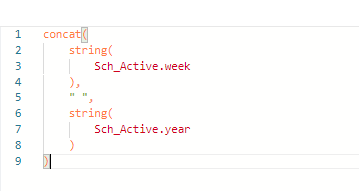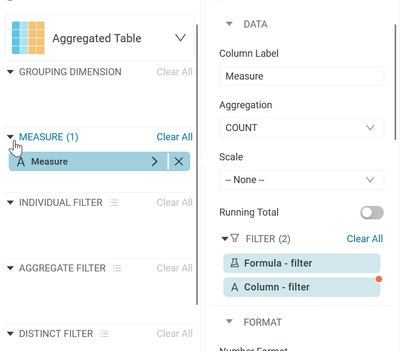cancel
Showing results for
Did you mean:

## How to count rows with several COUNTIFS conditions (multiple columns).. and moreRanger

Hello there,

As my adventure with Incorta continues, more deadly problems approaches on my road to glory :). So I’m stuck, I'm trying to count several values with various of conditions.

Normally in Excel I'm using such function :

=ROWS(UNIQUE(FILTER(Tabel1[WEEK]:Tabela1[User];(Tabel1[WEEK]=B51)*(Tabel1[YEAR]=\$A\$2))))

Above function counts my number of rows for distinct value of columns [User] that is within the range of the corresponding year and week.

=ROWS(UNIQUE(FILTER(Tabel1[WEEK]:Tabela1[User];(Tabel1[week last seen]=B29)*(Tabel1[week]=B29)*(Tabel1[Model]="CAR")*(Tabela1[year]=\$A\$3))))

Above function counts my number of rows for distinct value of columns [User] that is within the range of the corresponding year and week, and also checks if the values from column [week last seen] = corresponding week, and last condition is that that row need to be in "CAR" group Tabel1[Model].

column [week last seen] = WEEKNUM([date of last seen]];21) (date in format 27.10.2021) - this function returns number of week of that date

Last function contains one more condition, that I can’t describe :

Tabel1[Model]= and in here should be all values except "CAR"

On my chart I want that column week&year would be my grouping dimension so I made formula build :And function I’m trying to made would be the measures.

Also I’m trying to figure out how to made functions that would work like :

=COUNTIFS(Tabel1[Year];B3;Tabel1[week];B2;Tabel1[Date];"OK")

=IF(COUNTIFS([User];[@[User]];[YEAR];[@YEAR];[WEEK];[@WEEK])<=3;"OK";"NOK")

=IF(AND(ISNUMBER([@[week last seen]]);WEEKNUM([@[week last seen]];21)=[@WEEK]);"OK";"NOK")

I’m newbe in Incorta, so would be really grateful for any help. TY

2 REPLIES 2Partner

On any aggregated measure you can drop one or more filters which will ( probably ) get you where you want to go.

This is a quite handy feature for comparing the same measure in different contexts.Ranger

Thx RADSr, but as that solution gives me a possibility to count some values from multiple if , then I have a problem how to made  % chart from that data, eg :

1 . function : =ROWS(UNIQUE(FILTER(Tabel1[WEEK]:Tabela1[User];(Tabel1[WEEK]=B51)*(Tabel1[YEAR]=\$A\$2)))) -  will show me total qty of users in Week/Year period - that I can do with Incorta tools - number

2 . function - =ROWS(UNIQUE(FILTER(Tabel1[WEEK]:Tabela1[User];(Tabel1[week last seen]=B29)*(Tabel1[week]=B29)*(Tabel1[Model]="CAR")*(Tabela1[year]=\$A\$3)))) - will show me total qty of users in Week/Year period that has a modell "CAR" - that I can do with Incorta tools -  again number

But know having those values on chart how to take qty from function 2 and divide it from qty from function 1 ? Function 2/Function1 = percent of total users that use "CAR"

In my opinion, the only solution is to use formula builder, and in it write the conditions of function 2 (amount) and divide by the conditions of function 1 (amount), and I have no idea how to write it 😕